# lsline

Add least-squares line to scatter plot

## Syntax

``lsline``
``lsline(ax)``
``h = lsline(___)``

## Description

example

````lsline` superimposes a least-squares line on each scatter plot in the current axes.`lsline` ignores data points that are connected with solid, dashed, or dash-dot lines (`'-'`, `'--'`, or `'.-'`) because it does not consider them to be scatter plots. To produce scatter plots, use the MATLAB® `scatter` and `plot` functions. ```

example

````lsline(ax)` superimposes a least-squares line on the scatter plot in the axes specified by `ax` instead of the current axes (`gca`).```

example

````h = lsline(___)` returns a column vector of least-squares line objects `h` using any of the previous syntaxes. Use `h` to modify the properties of a specific least-squares line after you create it. For a list of properties, see Line Properties.```

## Examples

collapse all

Generate three sets of sample data and plot each set on the same figure.

```x = 1:10; rng default; % For reproducibility figure; y1 = x + randn(1,10); scatter(x,y1,25,'b','*') hold on y2 = 2*x + randn(1,10); plot(x,y2,'mo') y3 = 3*x + randn(1,10); plot(x,y3,'rx:')```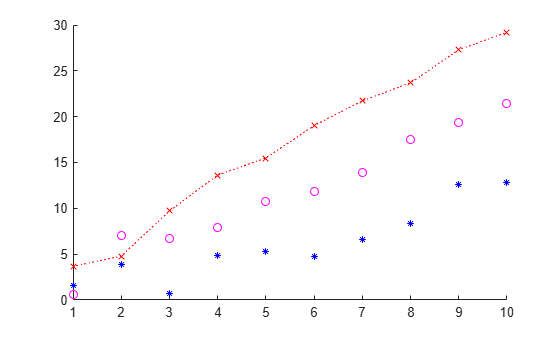Add a least-squares line for each set of sample data.

`lsline`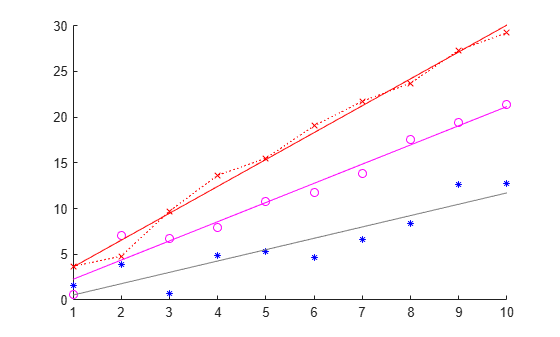Define the x-variable and two different y-variables to use for the plots.

```rng default % For reproducibility x = 1:10; y1 = x + randn(1,10); y2 = 2*x + randn(1,10);```

Define `ax1` as the top half of the figure, and `ax2` as the bottom half of the figure. Create the first scatter plot on the top axis using `y1`, and the second scatter plot on the bottom axis using `y2`.

```figure ax1 = subplot(2,1,1); ax2 = subplot(2,1,2); scatter(ax1,x,y1) scatter(ax2,x,y2)```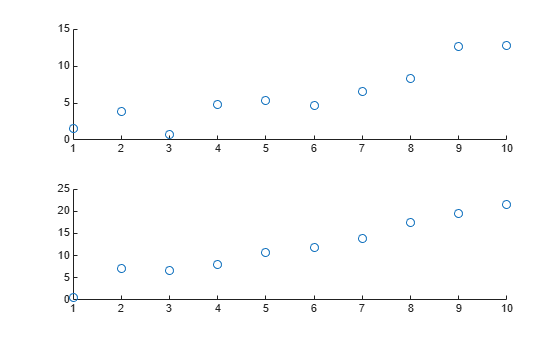Superimpose a least-squares line on the top plot, and a reference line at the mean of the `y2` values in the bottom plot.

```lsline(ax1) % This is equivalent to refline(ax1) mu = mean(y2); refline(ax2,[0 mu])```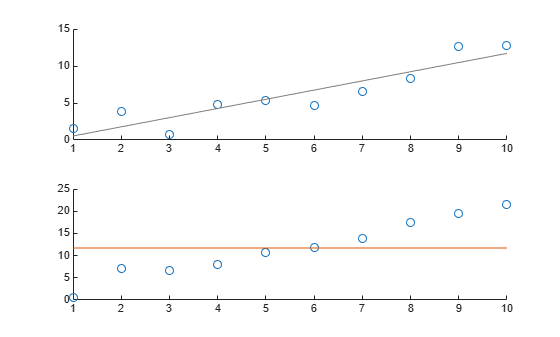Define the x-variable and two different y-variables to use for the plots.

```rng default % For reproducibility x = 1:10; y1 = x + randn(1,10); y2 = 2*x + randn(1,10);```

Define `ax1` as the top half of the figure, and `ax2` as the bottom half of the figure. Create the first scatter plot on the top axis using `y1`, and the second scatter plot on the bottom axis using `y2`.

```figure ax1 = subplot(2,1,1); ax2 = subplot(2,1,2); scatter(ax1,x,y1) scatter(ax2,x,y2)```

Superimpose a least-squares line on the top plot. Then, use the least-squares line object `h1` to change the line color to red.

```h1 = lsline(ax1); h1.Color = 'r';```

Superimpose a least-squares line on the bottom plot. Then, use the least-squares line object `h2` to increase the line width to 5.

```h2 = lsline(ax2); h2.LineWidth = 5;```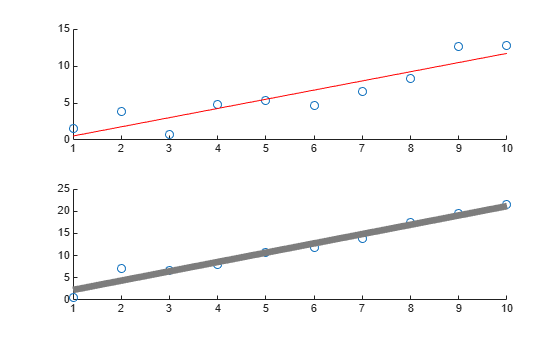## Input Arguments

collapse all

Target axes, specified as an `axes` object. If you do not specify the axes and if the current axes are Cartesian axes, then the `lsline` function uses the current axes.

## Output Arguments

collapse all

One or more least-squares line objects, returned as a scalar or a vector. These objects are unique identifiers, which you can use to query and modify properties of a specific least-squares line. For a list of properties, see Line Properties.